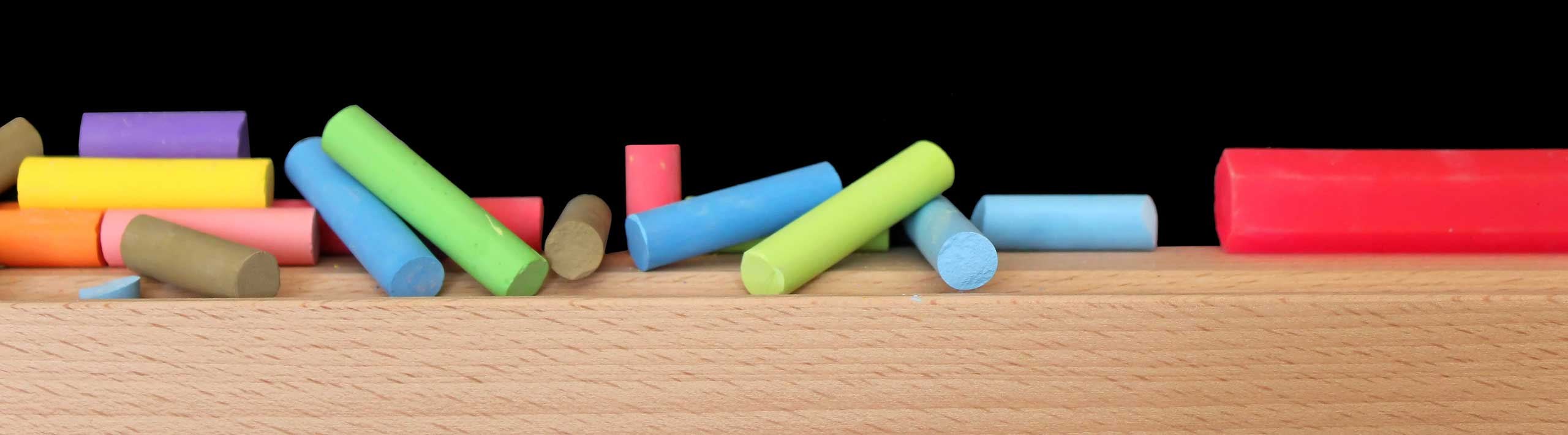# Solve 3 by 3 System Using Inverse Matrix - Calculators

This calculator solves a 3 by 3 systems of equations using the method of the inverse matrix.
The system of equations is written as

A X = K

where A is a 3 by 3 matrix whose elements are the coefficients of the unknown x, y and z in the system of equations, X is the 3 by 1 matrix whose elements are the unknown x, y and z and K is the 3 by 1 matrix whose elements are the constant terms on the right side of the equations. The solution is given by

X = A-1K
where A-1 is the inverse matrix of matrix A.

## Use the Calculator to Solve System

Enter the coefficients in the cells of the 3 by 3 matrix.(decimal, fraction of the form a/b and whole numbers as shown in the example below, are accepted), enter also the constant coefficients of the system on the right side, then press "Solve".

 A X = K -1 x    +    -3 y    +    6    z = -19 2 x    +    3/2 y    +    -5   z = 15 -4 x    +    -1 y    +    2.5    z = -11 Solution X = A-1 K x = = y = � = z = =